Next: Bibliography Up: The Meaning of Einstein's Previous: Spatial Curvature

The Mathematical Details

To see why equation (2) is equivalent to the usual formulation of Einstein's equation, we need a bit of tensor calculus. In particular, we need to understand the Riemann curvature tensor and the geodesic deviation equation. For a detailed explanation of these, the reader must turn to some of the texts in the bibliography. Here we briefly sketch the main ideas.

When spacetime is curved, the result of parallel transport depends on the path taken. To quantify this notion, pick two vectors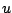and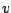at a point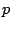in spacetime. In the limit where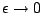, we can approximately speak of a 'parallelogram' with sides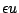and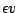. Take another vector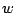atand parallel transport it first alongand then alongto the opposite corner of this parallelogram. The result is some vector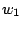. Alternatively, parallel transportfirst alongand then along. The result is a slightly different vector,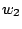: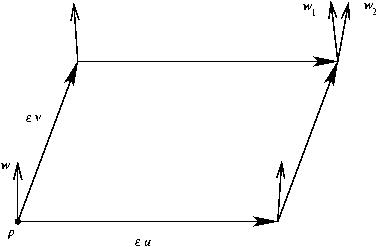The limit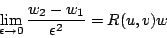(5)

is well-defined, and it measures the curvature of spacetime at the point. In local coordinates we can write it as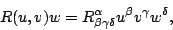where as usual we sum over repeated indices. The quantity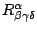is called the 'Riemann curvature tensor'.

We can use this tensor to compute the relative acceleration of nearby particles in free fall if they are initially at rest relative to one another. Consider two freely falling particles at nearby pointsand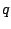. Letbe the velocity of the particle at, and letbe the vector fromto. Since the two particles start out at rest relative to one other, the velocity of the particle atis obtained by parallel transportingalong.

Now let us wait a short while. Both particles trace out geodesics as time passes, and at time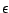they will be at new points, say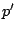and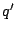. The pointis displaced fromby an amount, so we get a little parallelogram, exactly as in the definition of the Riemann curvature: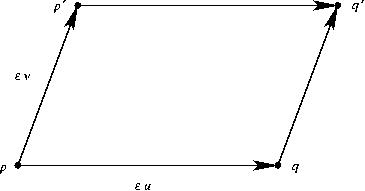Next let us compute the new relative velocity of the two particles. To compare vectors we must carry one to another using parallel transport. Let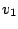be the vector we get by taking the velocity vector of the particle atand parallel transporting it toalong the top edge of our parallelogram. Let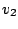be the velocity of the particle at. The difference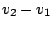is the new relative velocity. Here is a picture of the whole situation: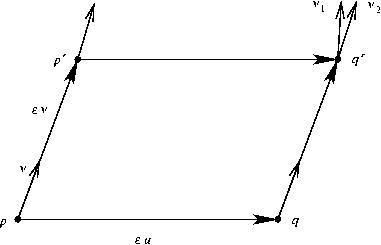The vectoris depicted as shorter thanfor purely artistic reasons.

It follows that over this passage of time, the average relative acceleration of the two particles is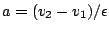. By equation (5),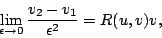so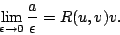This is called the 'geodesic deviation equation'. From the definition of the Riemann curvature it is easy to see that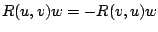, so we can also write this equation as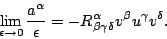(6)

Using this equation we can work out the second time derivative of the volume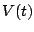of a small ball of test particles that start out at rest relative to each other. For this we must letrange over an orthonormal basis of tangent vectors, and sum the 'outwards' component of acceleration for each one of these. By equation (6) this gives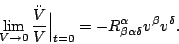In terms of the 'Ricci tensor'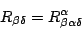we may write this as: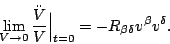In local inertial coordinates where the ball starts out at rest we have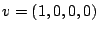, so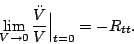(7)

In short, the Ricci tensor says how our ball of freely falling test particles starts changing in volume. The Ricci tensor only captures some of the information in the Riemann curvature tensor. The rest is captured by something called the 'Weyl tensor', which says how any such ball starts changing in shape. The Weyl tensor describes tidal forces, gravitational waves and the like.

Now, Einstein's equation in its usual form says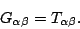Here the right side is the stress-energy tensor, while the left side, the 'Einstein tensor', is just an abbreviation for a quantity constructed from the Ricci tensor: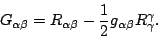Thus Einstein's equation really says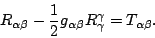(8)

This implies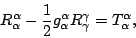but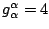, so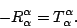Plugging this in equation (8), we get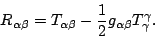(9)

This is equivalent version of Einstein's equation, but with the roles of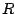andswitched! The good thing about this version is that it gives a formula for the Ricci tensor, which has a simple geometrical meaning.

Equation (9) will be true if any one component holds in all local inertial coordinate systems. This is a bit like the observation that all of Maxwell's equations are contained in Gauss's law and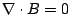. Of course, this is only true if we know how the fields transform under change of coordinates. Here we assume that the transformation laws are known. Given this, Einstein's equation is equivalent to the fact that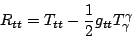(10)

in every local inertial coordinate system about every point. In such coordinates we have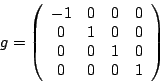(11)

so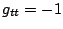and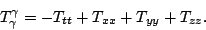Equation (10) thus says that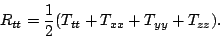By equation (7), this is equivalent to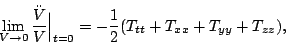as desired.

Next: Bibliography Up: The Meaning of Einstein's Previous: Spatial Curvature

© 2006 John Baez and Emory Bunn